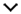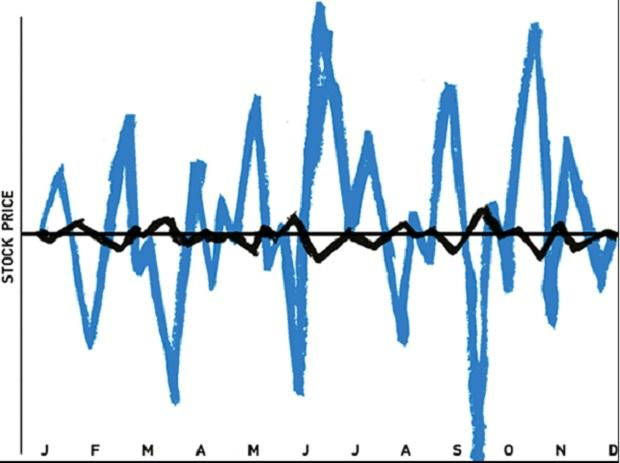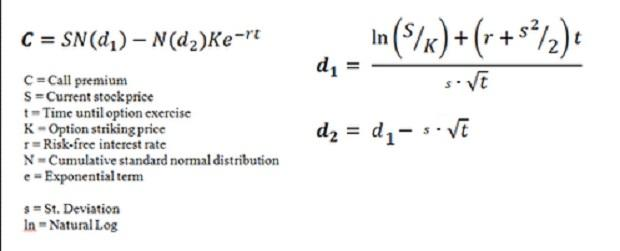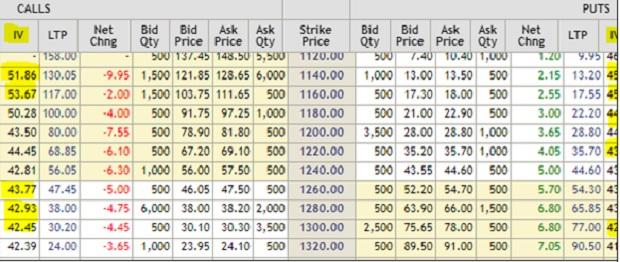You are here: Home » Markets » News
••Web Exclusive

How to measure and interpret implied volatility for trading options

Implied volatility is a measure of implied risk that traders are imputing in the option price

Sneha Seth | Angel Broking  |  MumbaiTipping point: Stick to US-focused funds despite volatility

When it comes to of options, it is slightly difficult to understand the concept offhand, unless you are able to understand a variety of related concepts. For example, it is essential to understand and the Black & Scholes Model for options valuation before you can apply IVs. Let us begin with first!Chart Source: Options Play Book
Volatility is crudely measures how much the stock price or index price is fluctuating. In the above chart, Blue line is more volatile than the black line. When it comes to volatility in equity markets, it is a measure of risk. But when it comes to options, volatility is positive for the value of options. Both the call option (right to buy) and the put option (right to sell) benefit from higher volatility. For that let us go back and delve a bit into the Black & Scholes model.

Black & Scholes model for option valuation

Black & Scholes is very complicated model that is hard to calculate manually but with an excel spread sheet, it can be managed in a jiffy. We can summarize the mathematical formula to calculate options value via Black & Scholes as under:If you feel that this formula looks extremely complicated, don’t fret over it. You can understand it as the outcome of five key factors viz.

• Spot price of the stock
• Options strike price
• (standard deviation)
• Time to expiry
• Yield on government bond

A change in any of these factors changes the value of the option. But the most important factor to understand is volatility. The volatility of the stock impacts the value of the call option and the put option positively. That means; greater the volatility, greater the chances of making profit for the buyer and hence greater the option value. That is why options tend to become more valuable in volatile times.

Moving over to implied volatility

To understand implied volatility, let us stay on with the Black & Scholes formula. In the Black and Scholes formula you use historical volatility and then use the above formula to calculate the value of an option. Then you decide whether the option is underpriced or overpriced. When you calculate the implied volatility, you do a bit of reverse engineering. You use the same formula but you don’t calculate option value. Instead you take the market price of the option as its intrinsic value and then work backward and calculate the volatility. This is the volatility that is implied in the option price and is called the We shall later see why this concept is so important and how to apply this in practice in option trading.

The whole purpose of understanding the concept of implied volatility of an option is to get some important cues on how to trade that option. Implied volatility is a measure of implied risk that traders are imputing in the option price. Let us look at the live option chain of Reliance Industries to understand IVs.Table Source: NSE
Each strike of Reliance has distinct IVs for calls and for puts. Normally, IVs are relevant only when there are sufficient volumes. Here is how you must go about interpreting IVs.

• From an options trader point of view, deep ITM IVs and deep OTM IVs don’t really matter. It is only the IVs of strike that are near to the spot price (ATM or Near-to-Money) that are really relevant for traders.
• Shifts in are normally a signal of how much risk traders are assigning to the stock. Normally, the representative is the mean of the ATM and near-to-money contracts. Rising IVs means are getting riskier and vice versa.
• Do low IVs imply bullishness? Firstly, the can sustain low volatility for some time and the IVs will need to converge toward the mean. Secondly, we have also seen volatility in puts going up when traders hedge too much and that leads to rise in value of puts and bearishness in the market.
• Traders often look at IV as a measure of market direction. It is not about bulls and bears but IV is purely about expected volatility. Increase in IV means that the expectation of volatility is going up.
• IVs are used for long Volatility (Long-Vol) and short volatility trades (Short-Vol). When you see IVs moving up it means expectations of volatility are going up and that is a good time to buy a straddle or strangle. Similarly, when IVs are drifting lower, it is time to play the (Short-Vol) trade by either selling straddles or strangles.
IV is one of the most important inputs for an options trader. Typically, options traders use a combination of cues from OI, PCR and IV to take create options positions.

Disclaimer: The above opinion is that of Ms. Sneha Seth (Derivatives Analyst - Angel Broking) & is for reference only.

First Published: Fri, June 07 2019. 07:47 IST